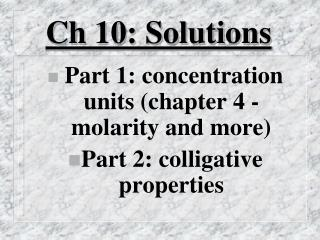DownloadDownload PresentationCh 10: Solutions

# Ch 10: Solutions

Télécharger la présentation## Ch 10: Solutions

- - - - - - - - - - - - - - - - - - - - - - - - - - - E N D - - - - - - - - - - - - - - - - - - - - - - - - - - -
##### Presentation Transcript

1. Ch 10: Solutions • Part 1: concentration units (chapter 4 - molarity and more) • Part 2: colligative properties

2. Part 1: calculations for preparing diluted solutions from more concentrated (note: we did this - the mols of solute stay the same)

3. Most difficult part: converting from one concentration unit to another (see. P. 260 for help on where to start)

4. Conc. of Solutes • A. Mass % = (mass solute) / (total mass solution) * 100% • ppm = mass% * 104 • ppb = mass % * 107

5. A. Mole Fraction: Xa = (no. mol A) / (total no. mol.)

6. Dissolve 12.0g methanol in 100g of water. What is the mole fraction of methanol?

7. B. Molality (m) = (no. mol solute) / (no. kg solvent)

8. Dissolve 12.0g methanol in 100g of water. What is the molality of methanol?

9. C. Molarity (M) = (no. mol solute) / (no. liters solution)

10. How do you prepare 35.0ml of 0.200 M aluminum nitrate from a 0.500 M solution?

11. What is the molality of a 0.200 M aluminum nitrate solution (d = 1.012g/mL)?

12. Solubility Principles • A. Nature of solute and solvent: most nonelectrolytes that are appreciably soluble in water involve hydrogen bonding.

13. A. Ex. Methanol, sugar, hydrogen peroxide.

14. Other nonelectrolytes tend to be more soluble in nonpolar or slightly polar solvents (benzene, toluene).

15. B. Effect of temperature: increase in T favors the endothermic process.

16. B. solid + water --> solution, DH is usually positive. Solubility increases with T.

17. B. gas + water --> solution, DH is usually negative. Solubility decreases with T.

18. C. Effect of pressure: negligible, except for gases, where solubility is directly proportional to the partial pressure of the gas (ex. carbonated soda).

19. C. Henry’s Law: Cg = kPg. • k = a constant involving the particular liquid/vapor system. • Cg = gas solubility in liquid • (solubility is directly proportional to pressure)

20. Henry’s Law The solubility of pure nitrogen in the blood at body temperature and 1 atm is 6.2 x 10-4M. If a diver breathes air (XN2 = 0.78) at a depth where the pressure is 2.5 atm, calculate the concentration of N2 in his blood?

21. Colligative Properties • For nonelectrolytes, these are properties that are dependent upon the concentration of solute particles rather than the type.

22. The principles hold true to within a few percent up to concentrations of 1 M. Above, there are large deviations. Why?

23. A.Vapor pressure lowered - adding a solute lowers the concentration of solvent molecules in the liquid phase and lowers the vapor pressure.

24. Ex. 0.10 M glucose solution has a vapor pressure that is 0.008 mm Hg less than pure water at 0oC.

25. Ex. 0.30 M glucose solution has a vapor pressure that is 0.024 mm Hg less than pure water at 0oC (linear relationship?).

26. Raoult’s Law: DPL = X2Po1, where X2 = mol fraction of the solute and Po1 = vapor pressure of the pure solvent.

27. A solution contains 82.0g of glucose in 322g of water. Calculate the vapor pressure lowering at 25oC (vpH2O=23.76 mm Hg)

28. B. Osmosis, Osmotic Pressure: water moves through a semi-permeable membrane from a region of high vapor pressure (high mol fraction) to a region of low vapor pressure.

29. p = MRT, where p is the osmotic pressure, M is the molar concentration, and R is the gas law constant. • (osmotic pressure - pressure required to stop osmosis)

30. Calculate the osmotic pressure at 15oC of a solution prepared by dissolving 50.0g of sucrose, in enough water to form one liter of solution.

31. C. Boiling Point elevation, freezing point depression. • 1. Results from vapor pressure lowering.

32. 2. For water solutions: • DTf = 1.86oC * m • DTb = 0.52oC*m.

33. Calculate the boiling and freezing points of a solution containing 20.0g of ethylene glycol (molar mass = 62.0g/mol) in 50.0g of water.

34. Suppose a solution is prepared by dissolving 0.100g of a nonelectrolyte in 1.00g of water. The freezing point is found to be -1.00oC. What is the molar mass of the solute?

35. Electrolytes: the colligative effects are greater due to the increased number of particles (ionic compounds dissolve into anions and cations).

36. Ex. DTf = 1.86oC*m*i • Where I is the number of moles of ions per mole of solute: • NaCl(s)  Na+(aq) + Cl-(aq), i=2 • CaCl2(s)  Ca2+(aq) + 2Cl-(aq), i=3

37. The emp. Form. for a solid is found to be (C5H4)n. A 0.527g sample is dissolved in 40.19g CHCl3 (kb = 3.63 kg.oC/mol), raising the b.p. of the solvent 0.392oC. What is the calculated MM, the molecular formula, & exact MM of the solid? Why the deviation?

38. A 2.0g sample of insulin isolated from beef pancreas is dissolved in water to 250.0ml of solution. The osmotic pressure is found to be 26.1mmHg at 30.0oC. What is the molecular weight of this insulin?